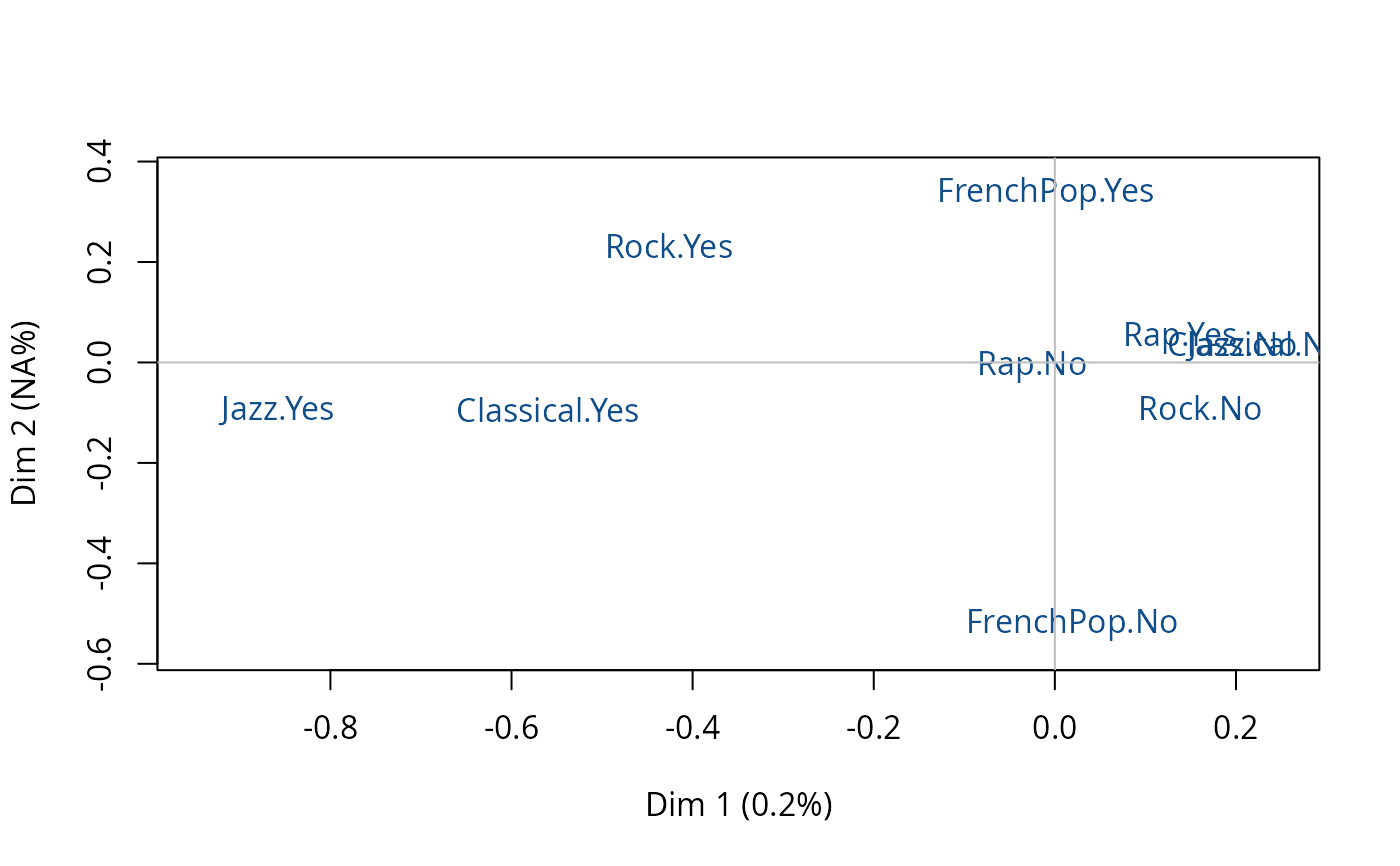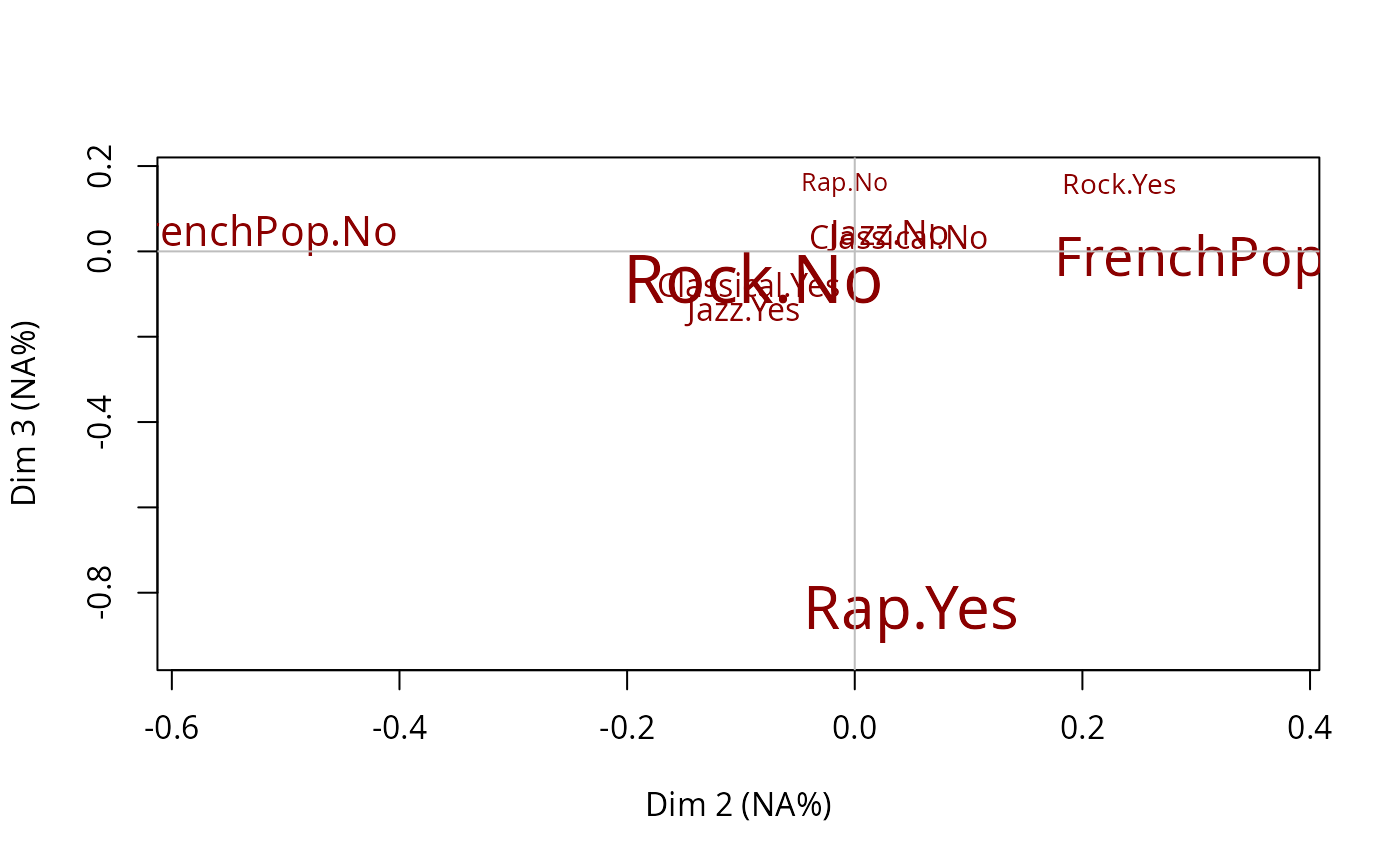Plots a standardized Multiple Correspondence Analysis (resulting from stMCA function), i.e. the clouds of individuals or categories.

# S3 method for stMCA
plot(x, type = "v", axes = 1:2, points = "all", threshold = 2.58, groups=NULL,
col = "dodgerblue4", app = 0, ...)

## Arguments

x

object of class stMCA

type

character string: 'v' to plot the categories (default), 'i' to plot individuals' points, 'inames' to plot individuals' names

axes

numeric vector of length 2, specifying the components (axes) to plot (c(1,2) is default)

points

character string. If 'all' all points are plotted (default); if 'besth' only those who are the most correlated to horizontal axis are plotted; if 'bestv' only those who are the most correlated to vertical axis are plotted; if 'best' only those who are the most coorelated to horizontal or vertical axis are plotted.

threshold

numeric value. V-test minimal value for the selection of plotted categories.

groups

only if x$call$input.mca = 'multiMCA', i.e. if the MCA standardized to x object was a multiMCA object. Numeric vector specifying the groups of categories to plot. By default, every groups of categories will be plotted

col

color for the points of the individuals or for the labels of the categories (default is 'dodgerblue4')

app

numerical value. If 0 (default), only the labels of the categories are plotted and their size is constant; if 1, only the labels are plotted and their size is proportional to the weights of the categories; if 2, points (triangles) and labels are plotted, and points size is proportional to the weight of the categories.

...

further arguments passed to or from other methods, such as cex, cex.main, ...

## Details

A category is considered to be one of the most correlated to a given axis if its test-value is higher then 2.58 (which corresponds to a 0.05 threshold).

## Author

Nicolas Robette

stMCA, textvarsup, conc.ellipse

## Examples

# standardized MCA of Music example data set
# controlling for age
## and then draws the cloud of categories.
data(Music)
junk <- c("FrenchPop.NA", "Rap.NA", "Rock.NA", "Jazz.NA", "Classical.NA")
mca <- speMCA(Music[,1:5], excl = junk)
stmca <- stMCA(mca, control = list(Music\$Age))
# cloud of categories
plot(stmca)# cloud of categories on dimensions 2 and 3
plot(stmca, axes = c(2,3), points = "best", col = "darkred", app = 1)